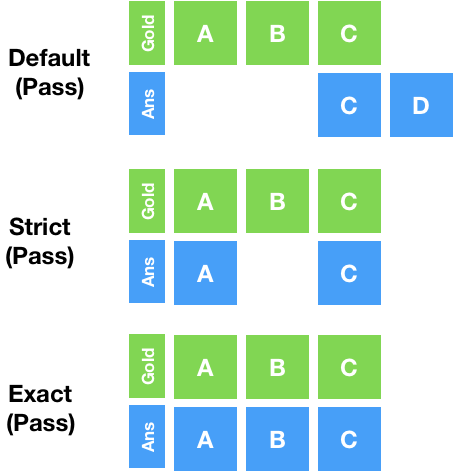Follow

# Test Question Matching

The default test question setting will pass a contributor as long as one of their answers matches one or more of your answers even if they've also selected a wrong answer. For example, if you select A and B as the correct answer, for a multiple checkbox question, a contributor will pass the test question if they select “A” or “B”. In this example, the contributor will be marked correct even if he/she selected a wrong answer. If you decide to be less lenient with test questions, you will want to use either of the following attributes:

`exact`

If set to,`"true"(exact="true")` the set of contributor responses must be identical to the set of pre-defined correct responses on a test question in order for that contributor's judgment to be considered correct. A contributor's response will not be considered correct if they include a response not found in the set of correct responses. For example, if responses "a", "b", and "c" are defined as correct in the test question data, then a contributor will only be correct if they submit "a", "b", and "c". Order does not matter.

Note: this setting will also attempt to match a test question even if it is left blank. For example, if you choose to not provide an answer for a set of checkboxes, the correct response with exact="true" will be none of the possible answers.

Example CML:

`<cml:checkboxes label="Sample checkboxes:" validates="required" exact="true"><cml:checkbox label="Checkbox 1" checked="true" /> <cml:checkbox label="Checkbox 2" /> <cml:checkbox label="Checkbox 3" /> </cml:checkboxes>`

`strict`

If set to `"true"(strict="true")`, every response submitted by a contributor must be included in the set of pre-defined correct responses on a test question in order for that contributor's judgment to be considered correct. Unlike `exact`, `strict` allows contributors to omit responses that are part of the test question set. For example, if responses "a", "b", and "c" are defined as correct in the test question data, then a contributor will be considered correct if they submit only "a" and "b".

`matcher`

Allows you to redefine how a contributor's responses to test questions are evaluated. Normally, a contributor's responses must exactly match that of the stored test question answers. This can be especially helpful when used with numeric or alpha test questions. This attribute can be set to the following:

• `not` - This will reverse the normal behavior so that a contributor must answer anything but the pre-defined test question answer to be considered correct. For example, if answer "a" was stored in the test question data and the contributor selects answer "b" (or anything other than "a"), the contributor would get the test question correct.
• `range` - This allows you to specify a range that a contributor response can fall between. Specifying a minimum and maximum range will allow a greater threshold of acceptability when contributors answer test questions that may have some variances. To define the minimum and maximum, this can be set within the test question interface, or within a data file with two headers named "column_name_min" and "column_name_max". Use of a number validator is recommended in conjunction with this matcher.
• `fuzzy` - this allows you to specify a fuzzy matching threshold for text inputs (including cml:text and cml:text_area). This options accepts a value between 0 and 1. The fuzzy matching level is computed based on the word level Levenshtein distance. If the matching level is equal or above the threshold, the response passes, otherwise it fails.

Example CML:

`<cml:checkboxes label="Sample checkboxes:" validates="required" matcher="not"> <cml:checkbox label="Checkbox 1" checked="true" /> <cml:checkbox label="Checkbox 2" /> <cml:checkbox label="Checkbox 3" /> </cml:checkboxes>`Fig. 1: Examples of how different test question matching parameters evaluate contributors